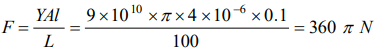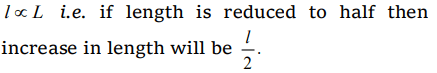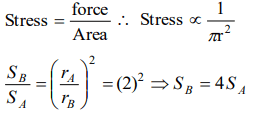## Mechanical Properties of Solids Questions and Answers Part-2

1. The material which practically does not show elastic after effect is
a) Copper
b) Rubber
c) Steel
d) Quartz

Explanation: Quartz

2. If the temperature increases, the modulus of elasticity
a) Decreases
b) Increases
c) Remains constant
d) Becomes zero

Explanation: Because due to increase in temperature intermolecular forces decreases.

3. A force F is needed to break a copper wire having radius R. The force needed to break a copper wire of radius 2R will be
a) F/2
b) 2F
c) 4F
d) F/4

Explanation:4. The relationship between Young's modulus Y, Bulk modulus K and modulus of rigidity $\eta$ is
a) $Y=\frac{9\eta K}{\eta+3 K}$
b) $Y=\frac{9Y K}{Y+ 3K}$
c) $Y=\frac{9\eta K}{3+ K}$
d) $Y=\frac{3\eta K}{9\eta+ K}$

Explanation:5. The diameter of a brass rod is 4 mm and Young's modulus of brass is $9\times10^{10}N\diagup m^{2}$  . The force required to stretch by 0.1% of its length is
a) $360\pi N$
b) 36 N
c) $144\pi \times10^{3} N$
d) $36\pi \times10^{5} N$

Explanation:6.If x longitudinal strain is produced in a wire of Young's modulus y, then energy stored in the material of the wire per unit volume is
a) $yx^{2}$
b) $2yx^{2}$
c) $\frac{1}{2}y^{2}x$
d) $\frac{1}{2}yx^{2}$

Explanation:7. In a wire of length L, the increase in its length is l. If the length is reduced to half, the increase in its length will be
a) l
b) 2l
c) $\frac{l}{2}$
d) None of the above

Explanation:8. The Young's modulus of a rubber string 8 cm long and density $1.5 kg\diagup m^{3}$   is $5\times10^{8} N\diagup m^{2}$  , is suspended on the ceiling in a room. The increase in length due to its own weight will be
a) $9.6\times10^{-5} m$
b) $9.6\times10^{-11} m$
c) $9.6\times10^{-3} m$
d) 9.6 m

Explanation:9. A and B are two wires. The radius of A is twice that of B. They are stretched by the some load. Then the stress on B is
a) Equal to that on A
b) Four times that on A
c) Two times that on A
d) Half that on AExplanation: Breaking force $\propto$ Area of cross section of wire i.e. load hold by the wire does not depend upon the length of the wire.